Question

# A bullet of mass 8 g is fired into a 1.2 kg ballistic pendulum (a block...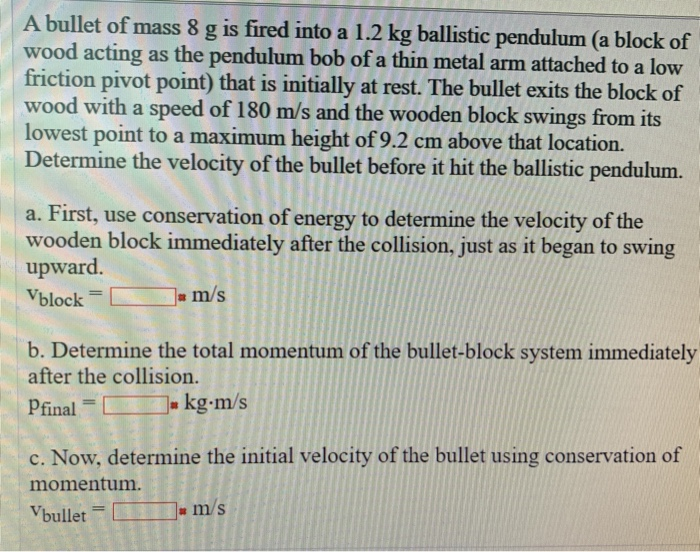A bullet of mass 8 g is fired into a 1.2 kg ballistic pendulum (a block of wood acting as the pendulum bob of a thin metal arm attached to a low friction pivot point) that is initially at rest. The bullet exits the block of wood with a speed of 180 m/s and the wooden block swings from its lowest point to a maximum height of 9.2 cm above that location. Determine the velocity of the bullet before it hit the ballistic pendulum. a. First, use conservation of energy to determine the velocity of the wooden block immediately after the collision, just as it began to swing upward. Vblock = m/s b. Determine the total momentum of the bullet-block system immediately after the collision. Pfinal = 0 . kg.m/s c. Now, determine the initial velocity of the bullet using conservation of momentum. Vbullet = m/s

using energy conservation between initial and final position of pendulum after collision

KEi + PEi = KEf + PEf

PEi = 0

KEf = 0, at highest point velocity will be zero.

KEi = PEf

0.5*m1*V1^2 = m1*g*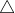h

V1 = final speed of block just after collision = sqrt (2*g*h)

V1 = Vblock = sqrt (2*9.81*0.092) = 1.344 m/sec

Part B.

Momentum of bullet-block system after collision will be:

Pf = m1*V1 + m2*V2

m2 = mass of bullet = 8 gm = 8*10^-3 kg

V2 = speed of bullet just after it exits the block = 180 m/sec

Pf = 1.2*1.344 + 8*10^-3*180 = 3.0528 kg-m/sec

Pf = 3.05 kg-m/sec = momentum of bullet-block system after the collision

Part C.

Now using momentum conservation before and after collision

Since this completely inelastic collision, So

Pi = Pf

m1*u1 + m2*u2 = Pf

u1 = initial speed of block = 0 m/sec

u2 = Initial speed of bullet = ?

So,

u2 = (Pf - m1*u1)/m2

u2 = (3.0528 - 1.2*0)/(8*10^-3)

u2 = 381.6 m/sec = Vbullet

#### Earn Coins

Coins can be redeemed for fabulous gifts.

Similar Homework Help Questions
• ### 1. A 35 g bullet is fired into the bob of a ballistic pendulum of mass...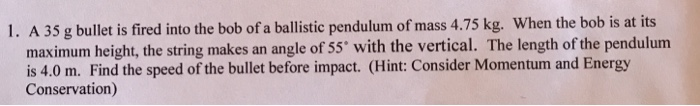1. A 35 g bullet is fired into the bob of a ballistic pendulum of mass 4.75 kg. When the bob is at its maximum height, the string makes an angle of 55 with the vertical. The length of the pendulum is 4.0 m. Find the speed of the bullet before impact. (Hint: Consider Momentum and Energy Conservation)

• ### Momentum, collisions, A 7.0 g bullet is fired into a 1.5 kg ballistic pendulum. The bullet...

A 7.0 g bullet is fired into a 1.5 kg ballistic pendulum. The bullet emerges from the block with a speed of 200 m/s, and the block rises to a maximum height of 9.2 cm.Find the initial speed of the bullet.

• ### Question 3 4 pts The ballistic pendulum consists of a block of dense material suspended from...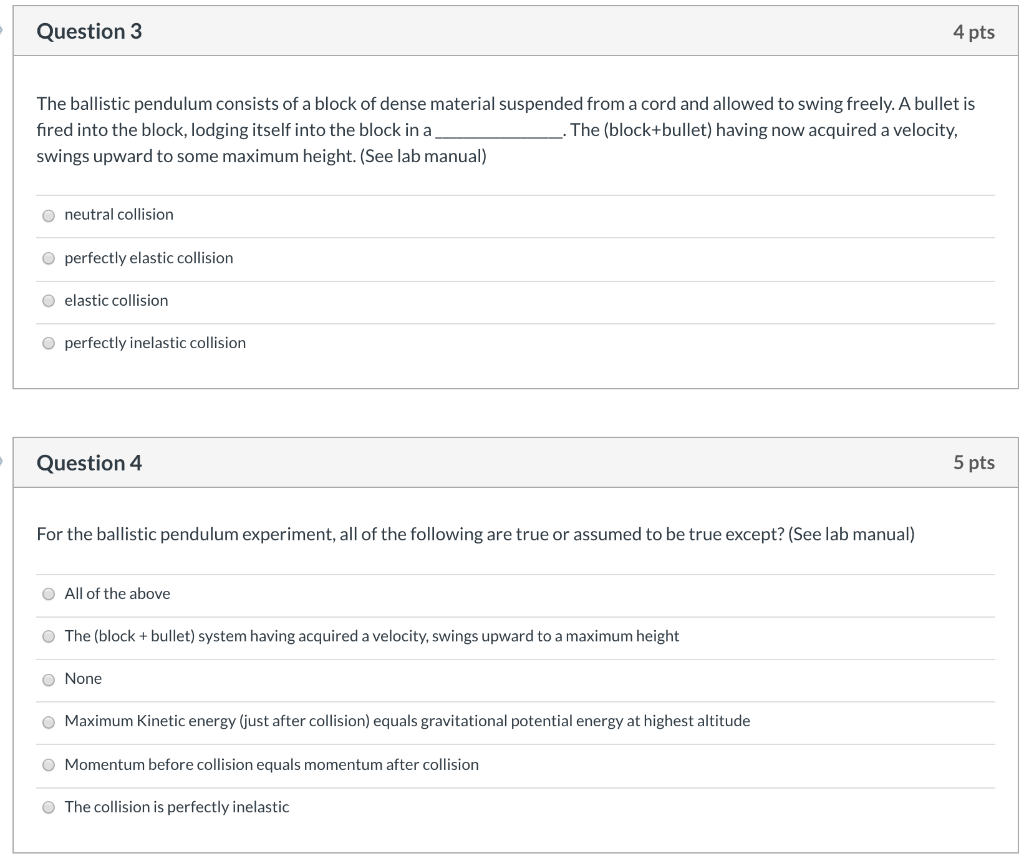Question 3 4 pts The ballistic pendulum consists of a block of dense material suspended from a cord and allowed to swing freely. A bullet is fired into the block, lodging itself into the block in a _. The (block+bullet) having now acquired a velocity, swings upward to some maximum height. (See lab manual) neutral collision perfectly elastic collision elastic collision perfectly inelastic collision Question 4 5 pts For the ballistic pendulum experiment, all of the following are true or...

• ### detail information please 11. The ballistic pendulum (see the figure below) is a device used to...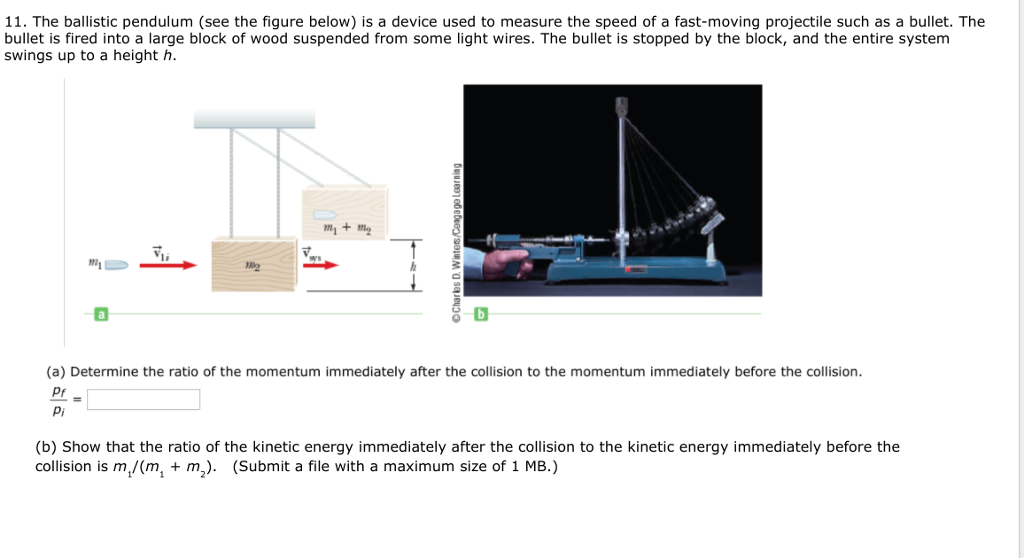detail information please 11. The ballistic pendulum (see the figure below) is a device used to measure the speed of a fast-moving projectile such as a bullet. The bullet is fired into a large block of wood suspended from some light wires. The bullet is stopped by the block, and the entire system swings up to a height h. m+m V1i 2 (a) Determine the ratio of the momentum immediately after the collision to the momentum immediately before the collision...

• ### Consider a projectile (0.1 kg) fired into a ballistic pendulum (0.5 kg, r_cm = 0.3 m),...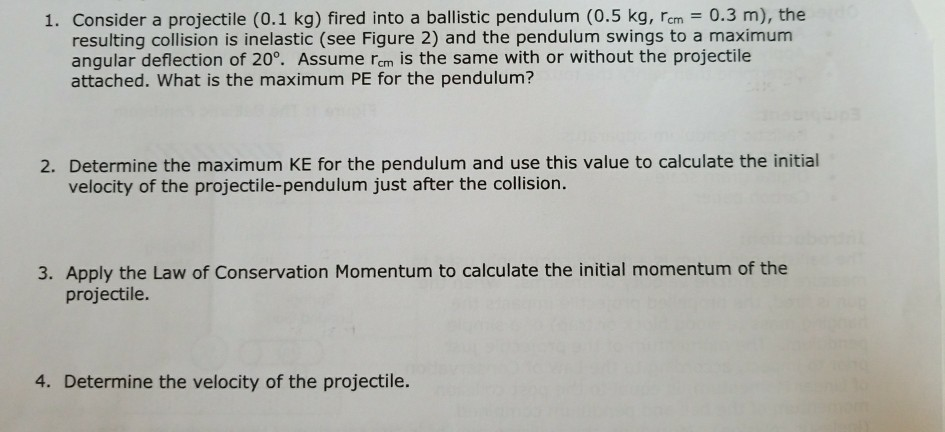Consider a projectile (0.1 kg) fired into a ballistic pendulum (0.5 kg, r_cm = 0.3 m), the resulting collision is inelastic (see Figure 2) and the pendulum swings to a maximum angular deflection of 20 degree. Assume r_cm is the same with or without the projectile attached. What is the maximum PE for the pendulum? Determine the maximum KE for the pendulum and use this value to calculate the initial velocity of the projectile-pendulum just after the collision. Apply the...

• ### A bullet of mass 10 g is fired horizontally into a block of wood of mass...

A bullet of mass 10 g is fired horizontally into a block of wood of mass 2 kg and suspended like a ballistic pendulum. The bullet sticks in the block and the impact causes the block to swing so that its center of gravity rises 0.1 m. Find the velocity of the bullet just before the impact. Can someone also explain the steps to me? I am very confused on all of this. Thank you

• ### mass One can measure the speed v of a bullet by using a ballistic pendulum. Let...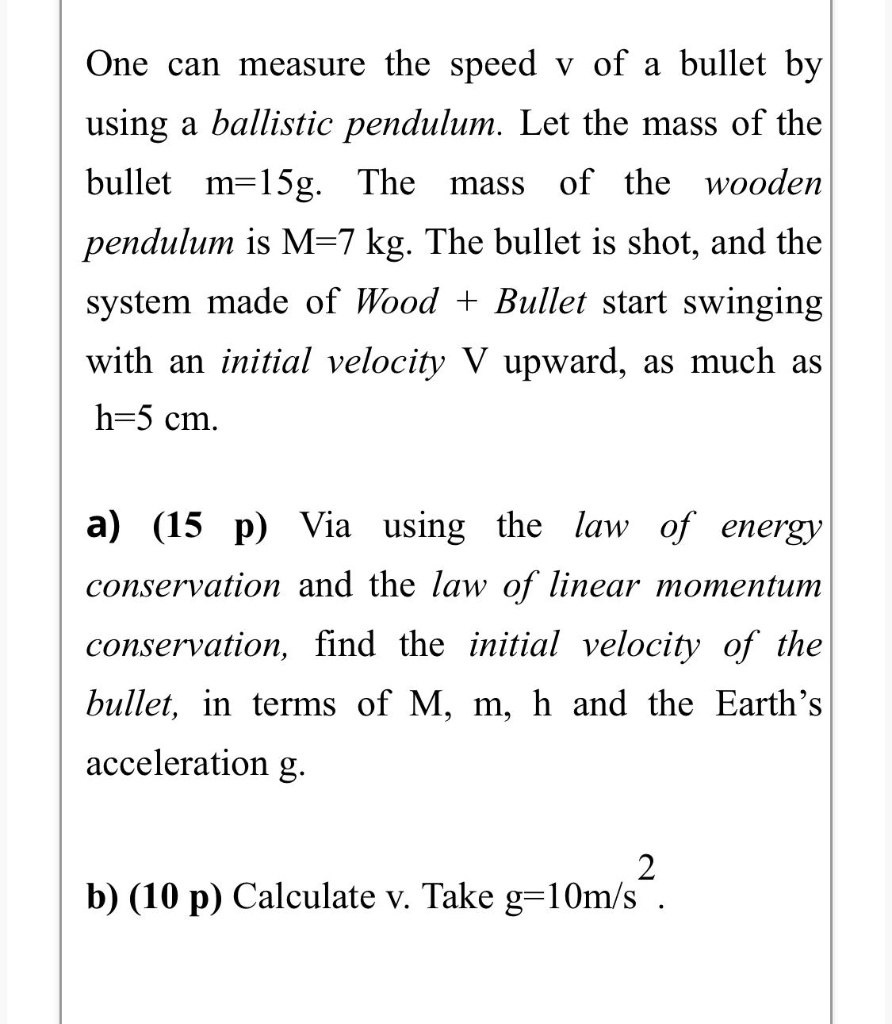mass One can measure the speed v of a bullet by using a ballistic pendulum. Let the mass of the bullet m=15g. The of the wooden pendulum is M=7 kg. The bullet is shot, and the system made of Wood + Bullet start swinging with an initial velocity V upward, as much as h=5 cm. a) (15 p) Via using the law of energy conservation and the law of linear momentum conservation, find the initial velocity of the bullet, in...

• ### One can measure the speed v of a bullet by using a ballistic pendulum. Let the...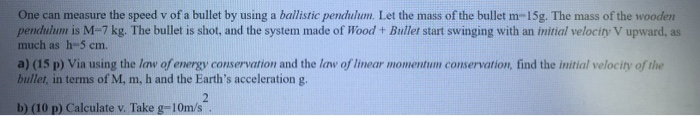One can measure the speed v of a bullet by using a ballistic pendulum. Let the mass of the bullet m-15g. The mass of the wooden pendulum is M-7 kg. The bullet is shot, and the system made of Wood + Bullet start swinging with an initial velocity V upward, as much as h-5 cm. a) (15 p) Via using the law of energy conservation and the law of linear momentum conservation, find the initial velocity of the bullet, in...

• ### In a ballistic pendulum an object of mass is fired with an initial speed at a pendulum bob. The bob has a mass, which...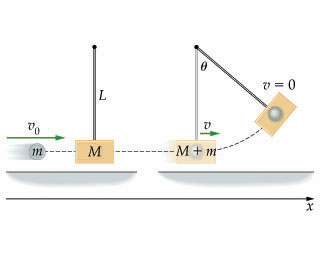In a ballistic pendulum, an object of mass m is fired with an initial speed v0 at a pendulum bob. The bob has a mass M, which is suspended by a rod of length L and negligible mass. After the collision, the pendulum and object stick together and swing to a maximum angular displacement θ as shown.A. Find an expression for v0, the initial speed of the fired object.Express your answer in terms of some or all of the variables m, M, L, and...

• ### 7.HW. A bullet is fired into a block of wood sitting on a block of ice....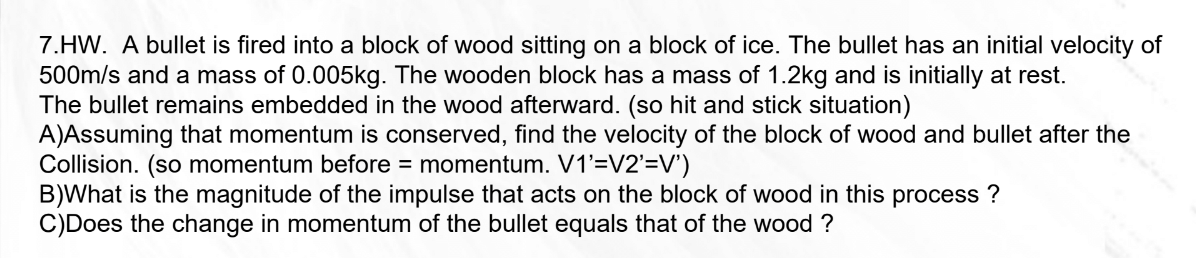7.HW. A bullet is fired into a block of wood sitting on a block of ice. The bullet has an initial velocity of 500m/s and a mass of 0.005kg. The wooden block has a mass of 1.2kg and is initially at rest. The bullet remains embedded in the wood afterward. (so hit and stick situation) A)Assuming that momentum is conserved, find the velocity of the block of wood and bullet after the Collision. (so momentum before = momentum. V1’=V2'=V') B)What...